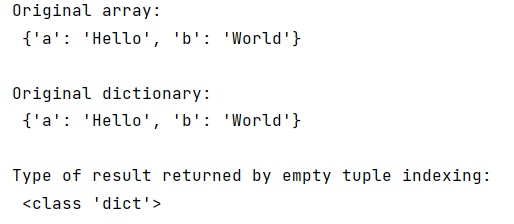# Recover dict from 0-d numpy array

Learn, how to recover dict from 0-d numpy array in Python?
Submitted by Pranit Sharma, on January 25, 2023

NumPy is an abbreviated form of Numerical Python. It is used for different types of scientific operations in python. Numpy is a vast library in python which is used for almost every kind of scientific or mathematical operation. It is itself an array which is a collection of various methods and functions for processing the arrays.

## Recovering dict from 0-d numpy array

Suppose that we are given some data in the form of a dictionary and we save the data of this dictionary in the form of a numpy array and on loading this data the type of data is ndarray. We need to recover this data as a dictionary.

0-d arrays can be indexed using the empty tuple, hence, we will create the NumPy array using an array object and pass the entire dictionary inside it. On indexing using the empty tuple, it will return the exact dictionary and we can also check its type.

Let us understand with the help of an example,

## Python code to recover dict from 0-d numpy array

```# Import numpy
import numpy as np

# Creating a numpy array
arr = np.array({'a':'Hello','b':'World'})

# Display original array
print("Original array:\n",arr,"\n")

# indexing array using empty tuple
res = arr[()]

# Display original dictionary
print("Original dictionary:\n",res,"\n")

# Check the type of result
print("Type of result returned by empty tuple indexing:\n",type(res))
```

Output: